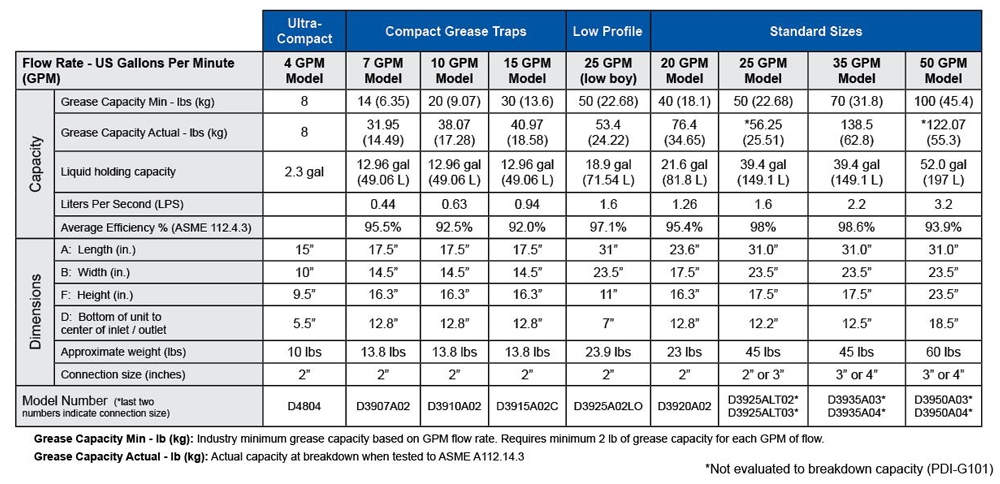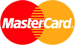Need help?   908-236-0277  CLICK TO CALL

How to determine the flow rate of your sink:

1. Measure your sinks. Calculate the capacity of the sink in cubic inches by multiplying length by width by depth. If you have multiple compartments or sinks, add the capacity of sinks together to find total cubic inches:
_____ x _____ x _____ = _______ Cubic Inches
Length x Width x Depth

2. Convert the capacity from total cubic inches to gallons per minute (GPM) by dividing by 231:
_______ Cubic Inches ÷ 231 = _______ GPM

3 . Adjust for displacement (displacement takes into consideration the actual usable capacity of your sink):
_______ GPM x 0.75 = _______ GPM Actual Drainage Load for sinks

Note:  Dishwashers can be connected to a Grease Trap only if allowed by local code.  Each dishwasher will require a seperate Grease Trap.  For floor drains and floor sinks take the volume of water produced by the number of hose bibs (i.e. 1.5 - 2.0 GPM per 3/4 inch faucet).
____________ + ___________
Actual Drainage Load for sinks + Other Appliances and sinks

5. Determine the flow rate and drainage period. Assume a 1 minute drainage period unless conditions permit a 2 minute drainage period. Drainage period is the actual time it takes to completely drain the fixture.
_______ GPM ÷ ________ drainage period = _________ GPM Total Actual Drainage Load
(1 or 2 min) (Flow Rate)

6. Select interceptor from the table below which corresponds with the flow rate calculated. Round up to the next largest size.The cart is empty

### Bestsellers